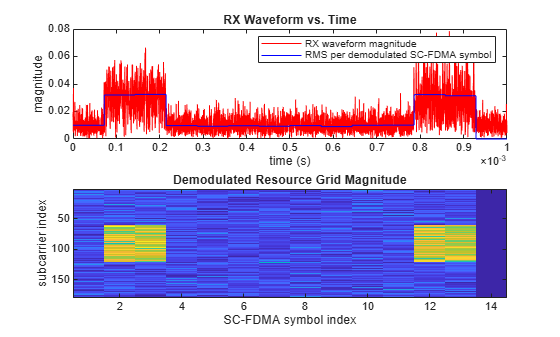## Syntax

``grid = lteSLSCFDMADemodulate(ue,waveform)``
``grid = lteSLSCFDMADemodulate(ue,waveform,cpfraction)``

## Description

example

````grid = lteSLSCFDMADemodulate(ue,waveform)` performs sidelink SC-FDMA demodulation of the input time-domain waveform for the specified UE settings structure. For more information, see Sidelink SC-FDMA Demodulation.```
````grid = lteSLSCFDMADemodulate(ue,waveform,cpfraction)` allows the specification of the starting waveform sample for demodulation as a fraction of the cyclic prefix.```

## Examples

collapse all

Perform sidelink SC-FDMA modulation of one subframe containing the sidelink synchronization signals and add noise at an SNR of 3.0 dB. The demodulator zeros the resource elements in the last SC-FDMA symbol. This behavior is consistent with the operation of the SC-FDMA modulator which does not modulate the last SC-FDMA symbol of the subframe. Plot the received waveform and the demodulated resource grid magnitude.

Create a UE settings structure.

```ue.NSLRB = 15; ue.CyclicPrefixSL = 'Normal'; ue.NSLID = 17;```

Populate the resource grid with PSSS and SSSS. Modulate the PSSS and SSSS.

```txgrid = lteSLResourceGrid(ue); txgrid(ltePSSSIndices(ue)) = ltePSSS(ue); txgrid(lteSSSSIndices(ue)) = lteSSSS(ue); [txwaveform,info] = lteSLSCFDMAModulate(ue,txgrid);```

Add AWGN with an SNR of 3.0 dB.

`rxwaveform = awgn(txwaveform,3.0,'measured');`

`rxgrid = lteSLSCFDMADemodulate(ue,rxwaveform);`

Calculate the RMS of each SC-FDMA symbol in the received resource grid.

`rms = sqrt(sum(abs((rxgrid./double(info.Nfft)).^2)));`

Plot the magnitude of the resulting time-domain waveform, overlaying the RMS for each SC-FDMA symbol after demodulation. Plot the demodulated resource grid magnitude.

```t = (0:size(rxwaveform,1))/info.SamplingRate; figure subplot(2,1,1) plot(t(1:end-1),abs(rxwaveform),'r') hold on n = cumsum([1 info.CyclicPrefixLengths + info.Nfft]); n = [n(1:end-1); n(2:end)]; rmsplot = repmat(rms,[2 1]); plot(t(n(:)),rmsplot(:),'b') xlabel('time (s)') ylabel('magnitude') title('RX Waveform vs. Time') legend('RX waveform magnitude','RMS per demodulated SC-FDMA symbol') subplot(2,1,2) imagesc(abs(rxgrid)) title('Demodulated Resource Grid Magnitude') xlabel('SC-FDMA symbol index') ylabel('subcarrier index')```## Input Arguments

collapse all

User equipment settings, specified as a parameter structure containing these fields:

Number of sidelink resource blocks, specified as an integer scalar from 6 to 110.

Example: `6`, which corresponds to a channel bandwidth of 1.4 MHz.

Data Types: `double`

Cyclic prefix length, specified as `'Normal'` or `'Extended'`.

Data Types: `char` | `string`

Data Types: `struct`

Sidelink SC-FDMA modulated waveform, specified as an NS-by-NT numeric matrix, where NS is the number of the time-domain samples and NT is the number of transmission antennas. NS = K × 30720 / 2048 × Nfft, where Nfft is the FFT size and K is the number of subframes in `waveform`.

For more information about the FFT size, see `lteSLSCFDMAInfo`.

Data Types: `double`
Complex Number Support: Yes

Fraction of cyclic prefix, specified as a numeric scalar from 0 to 1. A value of `0` represents the start of the cyclic prefix and a value of `1` represents the end of the cyclic prefix. The default value is `0.55` which assumes for the default level of windowing in the `lteSLSCFDMAModulate` function.

Data Types: `double`

## Output Arguments

collapse all

Resource element grid, returned as an NSC-by-NSYM-by-NT numeric array. NSC is 12 × `NSLRB` subcarriers. NSYM is a multiple of the number of SC-FDMA symbols in a subframe (14 for normal cyclic prefix and 12 for extended cyclic prefix).  NT is the number of antenna ports. `grid` defines the RE allocation across one or more subframes. Multiple subframes are defined by concatenation across the columns (second dimension).

Each antenna plane in `grid` is SC-FDMA modulated, resulting in the columns of `waveform`, as described in Represent Resource Grids.

Data Types: `double`
Complex Number Support: Yes

collapse all

Sidelink SC-FDMA demodulation recovers the received subcarrier values by performing one FFT operation per received sidelink SC-FDMA symbol. The recovered subcarrier values are used to construct each column of the output resource array grid. The FFT is positioned partway through the cyclic prefix, to account for some channel delay spread while avoiding the overlap between adjacent SC-FDMA symbols. The input FFT is also shifted by half of one subcarrier. The position of the FFT chosen in the function avoids the SC-FDMA symbol overlapping used in the `lteSLSCFDMAModulate` function. Because the FFT is performed away from the original zero-phase point on the transmitted subcarriers, `lteSLSCFDMADemodulate` applies a phase correction to each subcarrier after the FFT.
• TS 36.211 specifies that for PSSCH (Section 9.3.6), PSCCH (9.4.6), PSDCH (9.5.6) and PSBCH (9.6.6), resource elements in the last SC-FDMA symbol within a subframe should be counted in the mapping process but not transmitted. The resource elements of the last SC-FDMA symbol in each subframe of the output resource array grid are set to zero by `lteSLSCFDMADemodulate`. This behavior is consistent with SC-FDMA modulation, performed by `lteSLSCFDMAModulate`.
• The sampling rate of the time-domain sidelink waveform must be the same as the rate used in the `lteSLSCFDMAModulate` function, for the specified number of resource blocks, NRB.
• The input `waveform` must be time aligned, such that the first sample is the first sample of the cyclic prefix of the first sidelink SC-FDMA symbol in a subframe.